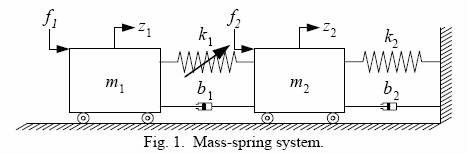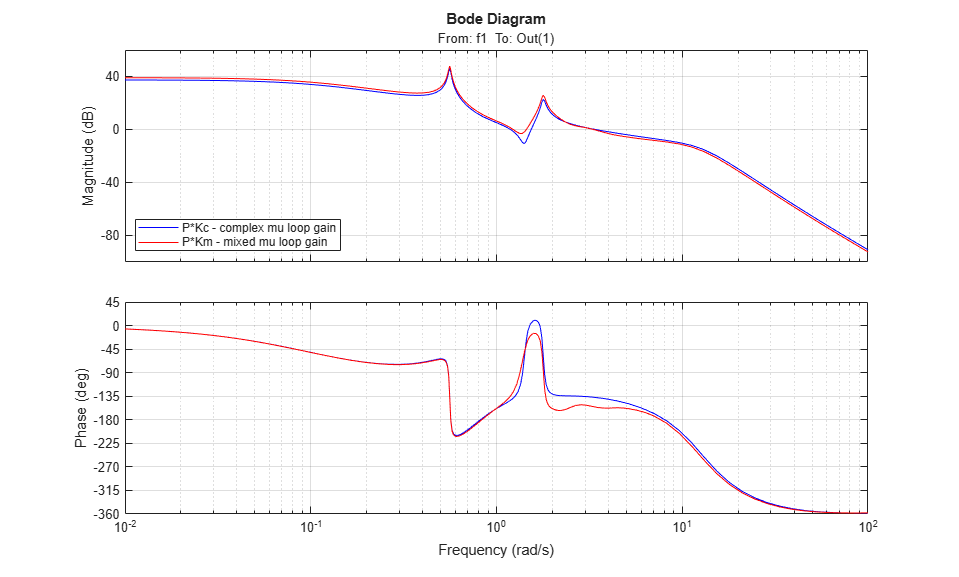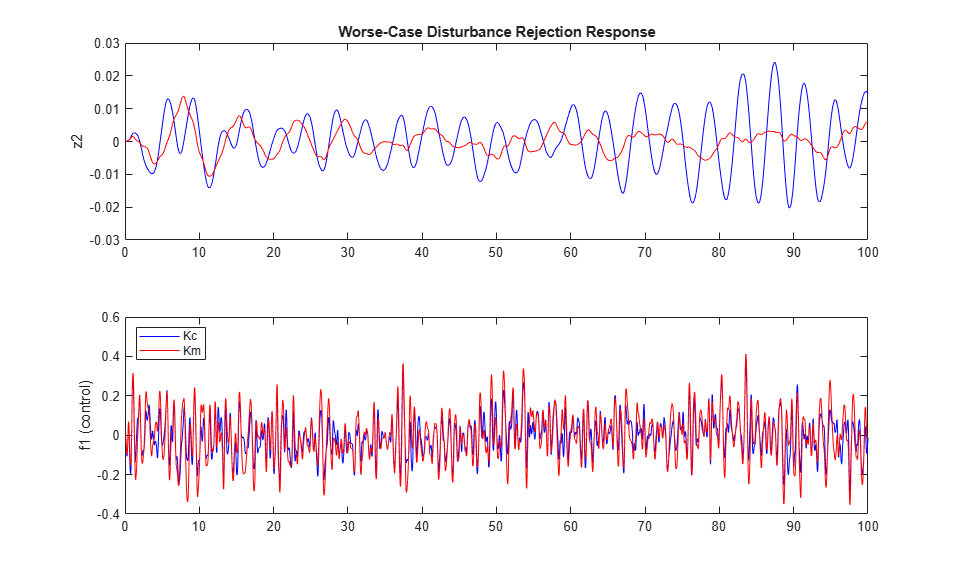# Control of a Spring-Mass-Damper System Using Mixed-Mu Synthesis

This example shows how to perform mixed-mu synthesis with the `musyn` command in the Robust Control Toolbox™. Here `musyn` is used to design a robust controller for a two mass-spring-damper system with uncertainty in the spring stiffness connecting the two masses. This example is taken from the paper "Robust mixed-mu synthesis performance for mass-spring system with stiffness uncertainty," D. Barros, S. Fekri and M. Athans, 2005 Mediterranean Control Conference.

### Performance Specifications

Consider the mass-spring-damper system in Figure 1. Spring `k2` and damper `b2` are attached to the wall and mass `m2`. Mass `m2` is also attached to mass `m1` through spring `k1` and damper `b1`. Mass 2 is affected by the disturbance force `f2`. The system is controlled via force `f1` acting on mass `m1`.Our design goal is to use the control force `f1` to attenuate the effect of the disturbance `f2` on the position of mass `m2`. The force `f1` does not directly act on mass `m2`, rather it acts through the spring stiffness `k1`. Hence any uncertainty in the spring stiffness `k1` will make the control problem more difficult. The control problem is formulated as:

• The controller measures the noisy displacement of mass `m2` and applies the control force `f1`. The sensor noise, `Wn`, is modeled as a constant 0.001.

• The actuator command is penalized by a factor 0.1 at low frequency and a factor 10 at high frequency with a crossover frequency of 100 rad/s (filter `Wu`).

• The unit magnitude, first-order coloring filter, `Wdist`, on the disturbance has a pole at 0.25 rad/s.

• The performance objective is to attenuate the disturbance on mass `m2` by a factor of 80 below 0.1 rad/s.

The nominal values of the system parameters are `m1=1`, `m2=2`, `k2=1`, `b1=0.05`, `b2=0.05`, and `k1=2`.

```Wn = tf(0.001); Wu = 10*tf([1 10],[1 1000]); Wdist = tf(0.25,[1 0.25],'inputname','dist','outputname','f2'); Wp = 80*tf(0.1,[1 0.1]); m1 = 1; m2 = 2; k2 = 1; b1 = 0.05; b2 = 0.05; ```

### Uncertainty Modeling

The value of spring stiffness `k1` is uncertain. It has a nominal value of 2 and its value can vary between 1.2 and 2.8.

```k1 = ureal('k1',2,'Range',[1.2 2.8]); ```

There is also a time delay `tau` between the commanded actuator force `f1` and its application to mass `m1`. The maximum delay is 0.06 seconds. Neglecting this time delay introduces a multiplicative error of `exp(-s*tau)-1`. This error can be treated as unmodeled dynamics bounded in magnitude by the high-pass filter `Wunmod = 2.6*s/(s + 40)`:

```tau = ss(1,'InputDelay',0.06); Wunmod = 2.6*tf([1 0],[1 40]); bodemag(tau-1,Wunmod,logspace(0,3,200)); title('Multiplicative Time-Delay Error: Actual vs. Bound') legend('Actual','Bound','Location','NorthWest') ```Construct an uncertain state-space model of the plant with the control force `f1` and disturbance `f2` as inputs.

```a1c = [0 0 -1/m1 1/m2]'*k1; a2c = [0 0 1/m1 -1/m2]'*k1 + [0 0 0 -k2/m2]'; a3c = [1 0 -b1/m1 b1/m2]'; a4c = [0 1 b1/m1 -(b1+b2)/m2]'; A = [a1c a2c a3c a4c]; plant = ss(A,[0 0;0 0;1/m1 0;0 1/m2],[0 1 0 0],[0 0]); plant.StateName = {'z1';'z2';'z1dot';'z2dot'}; plant.OutputName = {'z2'}; ```

Add the unmodeled delay dynamics at the first plant input.

```Delta = ultidyn('Delta',[1 1]); plant = plant * append(1+Delta*Wunmod,1); plant.InputName = {'f1','f2'}; ```

Plot the Bode response from f1 to z2 for 20 sample values of the uncertainty. The uncertainty on the value of k1 causes fluctuations in the natural frequencies of the plant modes.

```bode(plant(1,1),{0.1,4}) ```### Control Design

We use the following structure for controller synthesis:Figure 2

Use `connect` to construct the corresponding open-loop interconnection `IC`. Note that `IC` is an uncertain model with uncertain variables `k1` and `Delta`.

```Wu.u = 'f1'; Wu.y = 'Wu'; Wp.u = 'z2'; Wp.y = 'Wp'; Wn.u = 'noise'; Wn.y = 'Wn'; S = sumblk('z2n = z2 + Wn'); IC = connect(plant,Wdist,Wu,Wp,Wn,S,{'dist','noise','f1'},{'Wp','Wu','z2n'}) ```
```IC = Uncertain continuous-time state-space model with 3 outputs, 3 inputs, 8 states. The model uncertainty consists of the following blocks: Delta: Uncertain 1x1 LTI, peak gain = 1, 1 occurrences k1: Uncertain real, nominal = 2, range = [1.2,2.8], 1 occurrences Type "IC.NominalValue" to see the nominal value, "get(IC)" to see all properties, and "IC.Uncertainty" to interact with the uncertain elements. ```

### Complex mu-Synthesis

You can use the command `musyn` to synthesize a robust controller for the open-loop interconnection `IC`. By default, `musyn` treats all uncertain real parameters, in this example `k1`, as complex uncertainty. Recall that `k1` is a real parameter with a nominal value of 2 and a range between 1.2 and 2.8. In complex mu-synthesis, it is replaced by a complex uncertain parameter varying in a disk centered at 2 and with radius 0.8. The plot below compares the range of k1 values when k1 is treated as real (red x) vs. complex (blue *).

```k1c = ucomplex('k1c',2,'Radius',0.8); % complex approximation % Plot 80 samples of the real and complex parameters k1samp = usample(k1,80); k1csamp = usample(k1c,80); plot(k1samp(:),0*k1samp(:),'rx',real(k1csamp(:)),imag(k1csamp(:)),'b*') hold on % Draw value ranges for real and complex k1 plot(k1.Nominal,0,'rx',[1.2 2.8],[0 0],'r-','MarkerSize',14,'LineWidth',2) the=0:0.02*pi:2*pi; z=sin(the)+sqrt(-1)*cos(the); plot(real(0.8*z+2),imag(0.8*z),'b') hold off % Plot formatting axis([1 3 -1 1]), axis square ylabel('Imaginary'), xlabel('Real') title('Real vs. complex uncertainty model for k1') ```Synthesize a robust controller `Kc` using complex mu-synthesis (treating `k1` as a complex parameter).

```[Kc,mu_c,infoc] = musyn(IC,1,1); ```
``` D-K ITERATION SUMMARY: ----------------------------------------------------------------- Robust performance Fit order ----------------------------------------------------------------- Iter K Step Peak MU D Fit D 1 2.954 2.455 2.483 16 2 1.146 1.145 1.155 18 3 1.086 1.086 1.09 18 4 1.084 1.083 1.085 18 5 1.085 1.084 1.085 18 Best achieved robust performance: 1.08 ```

Note that `mu_c` exceeds 1 so the controller `Kc` fails to robustly achieve the desired performance level.

### Mixed-Mu Synthesis

Mixed-mu synthesis accounts for uncertain real parameters directly in the synthesis process. Enable mixed-mu synthesis by setting the `MixedMU` option to `'on'`.

```opt = musynOptions('MixedMU','on'); [Km,mu_m] = musyn(IC,1,1,opt); ```
``` DG-K ITERATION SUMMARY: ------------------------------------------------------------------- Robust performance Fit order ------------------------------------------------------------------- Iter K Step Peak MU DG Fit D G 1 2.954 2.081 2.371 16 8 2 1.673 1.432 1.675 14 8 3 0.9628 1.022 1.289 20 8 4 0.9163 0.9425 0.9871 20 8 5 0.896 0.9178 0.9747 20 8 6 0.8932 0.9047 0.9201 20 8 7 0.8883 0.8957 0.9312 20 8 8 0.8839 0.8877 0.916 18 8 9 0.885 0.8846 0.9074 16 8 10 0.8826 0.8849 0.9352 18 8 Best achieved robust performance: 0.885 ```

Mixed-mu synthesis is able to find a controller that achieves the desired performance and robustness objectives. A comparison of the open-loop responses shows that the mixed-mu controller `Km` gives less phase margin near 3 rad/s because it only needs to guard against real variations of `k1`.

```clf % Note: Negative sign because interconnection in Fig 2 uses positive feedback bode(-Kc*plant.NominalValue(1,1),'b',-Km*plant.NominalValue(1,1),'r',{1e-2,1e2}) grid legend('P*Kc - complex mu loop gain','P*Km - mixed mu loop gain','location','SouthWest') ```### Worst-Case Analysis

A comparison of the two controllers indicates that taking advantage of the "realness" of `k1` results in a better performing, more robust controller.

To assess the worst-case closed-loop performance of `Kc` and `Km`, form the closed-loop interconnection of Figure 2 and use the command `wcgain` to determine how large the disturbance-to-error norm can get for the specified plant uncertainty.

```clpKc = lft(IC,Kc); clpKm = lft(IC,Km); [maxgainKc,badpertKc] = wcgain(clpKc); maxgainKc ```
```maxgainKc = struct with fields: LowerBound: 2.1819 UpperBound: 2.1862 CriticalFrequency: 1.4308 ```
```[maxgainKm,badpertKm] = wcgain(clpKm); maxgainKm ```
```maxgainKm = struct with fields: LowerBound: 0.8833 UpperBound: 0.8844 CriticalFrequency: 0.1481 ```

The mixed-mu controller `Km` has a worst-case gain of 0.88 while the complex-mu controller `Kc` has a worst-case gain of 2.2, or 2.5 times larger.

### Disturbance Rejection Simulations

To compare the disturbance rejection performance of `Kc` and `Km`, first build closed-loop models of the transfer from input disturbance `dist` to `f2`, `f1`, and `z2` (position of the mass `m2`)```Km.u = 'z2'; Km.y = 'f1'; clsimKm = connect(plant,Wdist,Km,'dist',{'f2','f1','z2'}); Kc.u = 'z2'; Kc.y = 'f1'; clsimKc = connect(plant,Wdist,Kc,'dist',{'f2','f1','z2'}); ```

Inject white noise into the low-pass filter `Wdist` to simulate the input disturbance `f2`. The nominal closed-loop performance of the two designs is nearly identical.

```t = 0:.01:100; dist = randn(size(t)); yKc = lsim(clsimKc.Nominal,dist,t); yKm = lsim(clsimKm.Nominal,dist,t); % Plot subplot(311) plot(t,yKc(:,3),'b',t,yKm(:,3),'r') title('Nominal Disturbance Rejection Response') ylabel('z2') subplot(312) plot(t,yKc(:,2),'b',t,yKm(:,2),'r') ylabel('f1 (control)') legend('Kc','Km','Location','NorthWest') subplot(313) plot(t,yKc(:,1),'k') ylabel('f2 (disturbance)') xlabel('Time (sec)') ```Next, compare the worst-case scenarios for `Kc` and `Km` by setting the plant uncertainty to the worst-case values computed with `wcgain`.

```clsimKc_wc = usubs(clsimKc,badpertKc); clsimKm_wc = usubs(clsimKm,badpertKm); yKc_wc = lsim(clsimKc_wc,dist,t); yKm_wc = lsim(clsimKm_wc,dist,t); subplot(211) plot(t,yKc_wc(:,3),'b',t,yKm_wc(:,3),'r') title('Worse-Case Disturbance Rejection Response') ylabel('z2') subplot(212) plot(t,yKc_wc(:,2),'b',t,yKm_wc(:,2),'r') ylabel('f1 (control)') legend('Kc','Km','Location','NorthWest') ```This shows that the mixed-mu controller `Km` significantly outperforms `Kc` in the worst-case scenario. By exploiting the fact that `k1` is real, the mixed-mu controller is able to deliver better performance at equal robustness.

### Controller Simplification

The mixed-mu controller `Km` has relatively high order compared to the plant. To obtain a simpler controller, use `musyn`'s fixed-order tuning capability. This uses `hinfstruct` instead of `hinfsyn` for the synthesis step. You can try different orders to find the simplest controller that maintains robust performance. For example, try tuning a fifth-order controller. Use the "RandomStart" option to run several mu-synthesis cycles, each starting from a different initial value of `K`.

```K = tunableSS('K',5,1,1); % 5th-order tunable state-space model opt = musynOptions('MixedMU','on','MaxIter',20,'RandomStart',2); rng(0), [CL,mu_f] = musyn(lft(IC,K),opt); ```
```=== Synthesis 1 of 3 ============================================ DG-K ITERATION SUMMARY: ------------------------------------------------------------------- Robust performance Fit order ------------------------------------------------------------------- Iter K Step Peak MU DG Fit D G 1 22.03 15 15.16 10 6 2 6.431 6.362 10.14 16 6 3 4.229 4.206 4.841 18 8 4 3.747 3.695 4.288 18 8 5 2.613 2.592 2.723 18 8 6 1.821 1.842 2.729 18 8 7 1.588 1.585 1.585 18 8 8 1.215 1.262 1.706 20 8 9 1.206 1.206 1.268 18 8 10 1.113 1.112 1.245 18 8 11 1.081 1.08 1.106 18 8 12 1.03 1.029 1.26 18 8 13 1.041 1.04 1.084 16 8 14 1.019 1.019 1.122 20 8 15 1.028 1.028 1.072 16 8 16 1.022 1.022 1.051 18 8 Best achieved robust performance: 1.02 === Synthesis 2 of 3 ============================================ DG-K ITERATION SUMMARY: ------------------------------------------------------------------- Robust performance Fit order ------------------------------------------------------------------- Iter K Step Peak MU DG Fit D G 1 3.076 2.029 2.683 16 8 2 1.668 1.655 1.663 18 8 3 1.177 1.176 1.274 18 8 4 1.052 1.052 1.118 18 8 5 1.021 1.02 1.142 16 8 6 1.032 1.032 1.037 18 8 7 1.015 1.015 1.053 18 8 Best achieved robust performance: 1.01 === Synthesis 3 of 3 ============================================ DG-K ITERATION SUMMARY: ------------------------------------------------------------------- Robust performance Fit order ------------------------------------------------------------------- Iter K Step Peak MU DG Fit D G 1 3.194 3.084 3.084 16 8 2 2.401 2.377 2.392 18 8 3 1.733 1.732 1.903 18 8 4 1.225 1.22 1.226 20 8 5 1.054 1.076 1.233 18 8 6 1.025 1.025 1.159 16 8 7 1.036 1.035 1.058 18 8 8 1.017 1.016 1.079 18 8 Best achieved robust performance: 1.02 ```

The best controller nearly delivers the desired robust performance (robust performance `mu_f` is close to 1). Compare the two controllers.

```clf, bode(Km,getBlockValue(CL,'K')) legend('Full order','5th order') ```